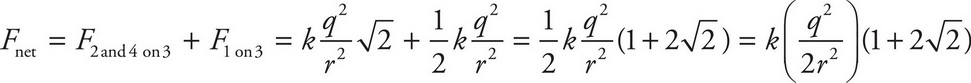# AP Physics 1 Question 372: Answer and Explanation

### Test Information

Question: 372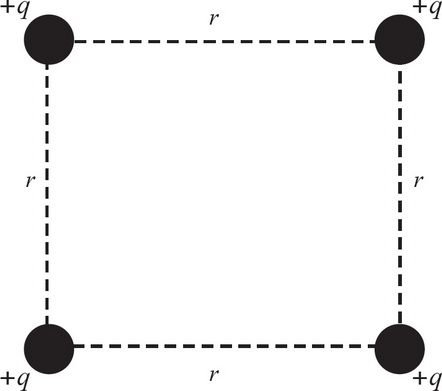10. In the figure above, four charges are arranged. If the magnitudes of all the charges q are all the same and the distance r between them is as shown above, what is the magnitude of the net force on the bottom right charge in terms of q, r, and k (where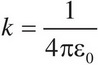)?

• A.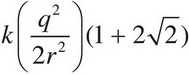• B.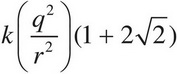• C.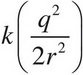• D.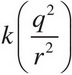Explanation:

A

Although you do not care about the direction of the net vector in the end, you do have to take orientation into account to find the magnitude. Number the charges from the top left and go clockwise as 1, 2, 3, and 4. Drawing out the different forces on the bottom right charge (charge 3), you get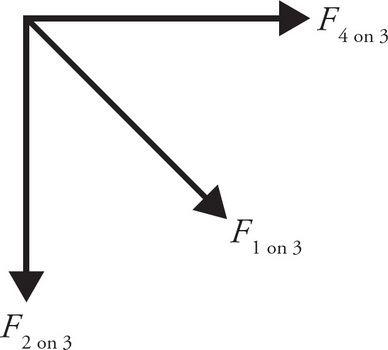Now solve each of these force vectors: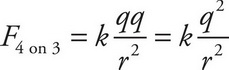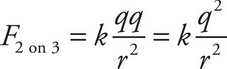These two are the simpler force vectors to solve. In order to solve charge 1's force on charge 3, the distance first needs to be solved. Because they are ordered in a square, using a diagonal creates a 45°-45°-90° triangle. The distance in this case is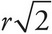. So,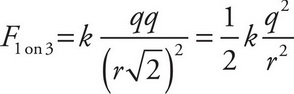First, combine the F4 on 3 with F2 on 3 to get an equivalent vector that will be in the same direction as F1 on 3,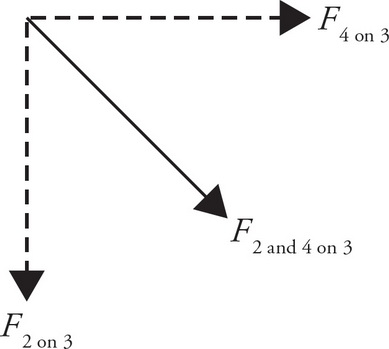They form a 45°-45°-90° triangle when laid tail-to-end so,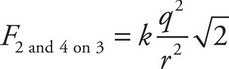This vector points in the same direction as F1 on 3, so to get the magnitude of the net force, simply add F2 and 4 on 3 with F1 on 3,